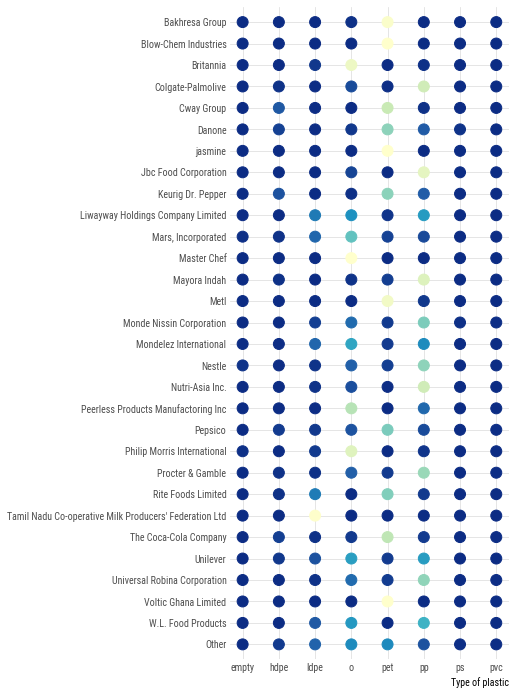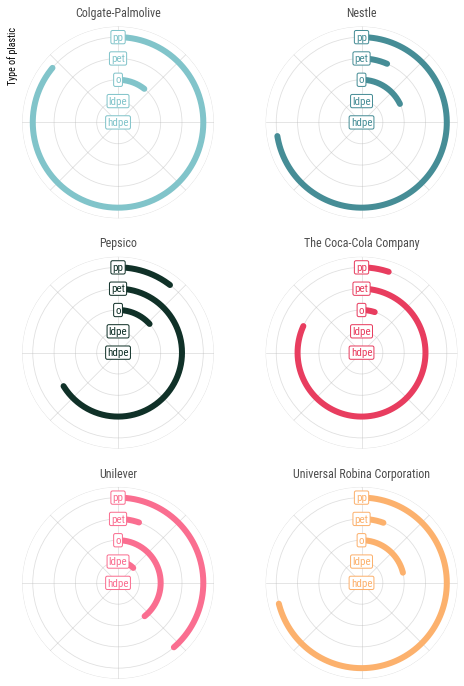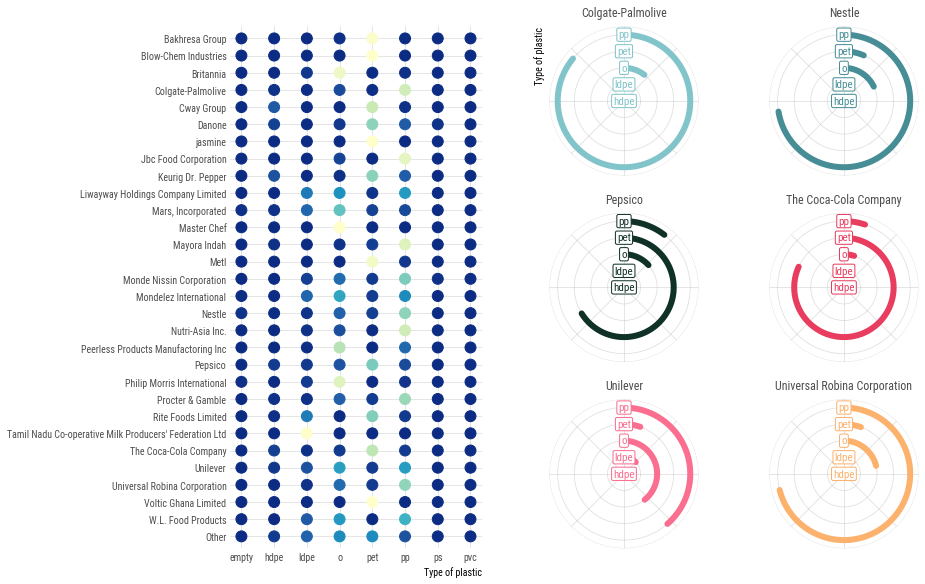# Heatmap and Radial Barchart with Matplotlib

This post shows how to create a vizualisation that is made of a heatmap and several radial barcharts arranged in a highly customized multi panel layout in `Python` and `Matplotlib`. This blogpost walks you through all the involved steps, from the data preparation to the final layout customizations.

This page showcases the work of Margaret Siple, built for the TidyTuesday initiative. You can find the original code on her GitHub repository here, written in R.

Thanks to him for accepting sharing his work here! Thanks also to Tomás Capretto who translated this work from R to Python! 🙏🙏

As a teaser, here is the plot we’re gonna try building:

## Import libraries

Matplotlib, Numpy, and Pandas power trio is all we're gonna use today.

``````import matplotlib.colors as mcolors
import matplotlib.pyplot as plt
import numpy as np
import pandas as pd``````

Before getting started with any data manipulation step, let's update Matplotlib's `rcParams` to use the Roboto Condensed font instead of the default one.

``plt.rcParams.update({"font.family": "Roboto Condensed"})``

## Load and prepare the data

The data for this article comes from Break Free from Plastic courtesy of Sarah Sauve. This guide uses the dataset released for the TidyTuesday initiative on the week of 2021-01-26. You can more information in the original announcement here. Thank you all for making this guide possible! 🙏🙏

The first step is to read the CSV file from the web.

``````path = "https://raw.githubusercontent.com/rfordatascience/tidytuesday/master/data/2021/2021-01-26/plastics.csv"

Then, we compute the total plastic waste for each type of plastic, by company and year.

``````types_of_plastic = ["empty", "hdpe", "ldpe", "o", "pet", "pp", "ps", "pvc"]
total_by_company = (
data
.groupby(["parent_company", "year"], dropna=True)[types_of_plastic]
.sum()
.reset_index()
)
total_by_company``````
parent_company year empty hdpe ldpe o pet pp ps pvc
0 "ESE" 2019 0.0 0.0 0.0 0.0 0.0 0.0 0.0 0.0
1 "Inko" noodles / Ð®Ñ„ÐºÐ° â€œÐ˜Ð½ÐºÐ¾" 2019 0.0 0.0 0.0 0.0 0.0 0.0 0.0 0.0
2 "Мелочи Жизни" (Ооо Ергопак) 2019 0.0 0.0 0.0 2.0 0.0 0.0 0.0 0.0
3 #ERROR! 2020 0.0 0.0 0.0 20.0 0.0 0.0 0.0 0.0
4 (Local Factory) 2020 0.0 0.0 0.0 0.0 9.0 20.0 0.0 0.0
... ... ... ... ... ... ... ... ... ... ...
11215 Ямуна 2019 0.0 0.0 0.0 3.0 0.0 0.0 0.0 0.0
11216 Янтар 2019 0.0 0.0 0.0 2.0 0.0 0.0 0.0 0.0
11217 Янтар (Сан Інбев Україна) 2019 0.0 0.0 0.0 2.0 0.0 0.0 0.0 0.0
11218 Янтарь 2019 0.0 0.0 0.0 1.0 0.0 0.0 0.0 0.0
11219 脆司令/Cui Siling 2019 0.0 0.0 0.0 1.0 0.0 0.0 0.0 0.0

11220 rows × 10 columns

The `get_top_n_data()` function gives the n companies that had the largest plastic waste. It performs several data manipulation steps that are explained with inline comments.

``````def get_top_n_data(data, n):
"""Create data frame with plastic values for the top-n companies and one Others group."""

# Analyses data from 2020 only
# Compute the total plastic waste per company
top_data = (
data
.query("year == 2020")
.melt(
id_vars=["parent_company", "year"],
value_vars=types_of_plastic,
var_name="type"
)
.groupby("parent_company")["value"]
.sum()
.reset_index()
)

# Use "Unbranded_unknown" for unbranded/unknown/error cases
top_data["parent_company"] = np.where(
top_data["parent_company"].isin(["Unbranded", "null", "NULL", "#ERROR!"]),
"Unbranded_unknown",
top_data["parent_company"]
)

# Create a list of top companies.
# This list contains the top n companies with largest plastic waste.
top_companies = list(top_data.sort_values("value", ascending=False)["parent_company"][:n])

# Create a lumped version of the company variable.
# If "parent_company" is one of the top companies, it gets its original name.
# Otherwise, it's labelled with "Other"
top_data["company_lumped"] = np.where(
top_data["parent_company"].isin(top_companies),
top_data["parent_company"],
"Other"
)

# Merge `top_data` with `total_by_company`
top_data = top_data.drop_duplicates(["parent_company", "company_lumped"])
top_data = top_data.merge(total_by_company, on="parent_company")

# Sum all types of plastic except from 'empty' into the "total" variable
top_data["total"] = top_data.loc[:, types_of_plastic[1:]].sum(axis=1)

## Heatmap

The first plot in the visualization we're trying to reproduce is a heatmap. This heatmap shows the proportion of plastic waste per plastic type for the top 30 companies.

Let's roll up our sleves and get to work on shaping this dataset!

``````top_thirty = get_top_n_data(total_by_company, n=30)

# For each company, compute the total waste per each type of plastic
top_thirty = (
top_thirty
.groupby("company_lumped")[types_of_plastic]
.sum()
.reset_index()
)

# Add a column with the sum of the plastic waste for all types of plastic
top_thirty["row_sum"] = top_thirty.loc[:, types_of_plastic].sum(axis=1)

# Divide the waste of each type of plastic by the total waste to get the proportion of waste for
# each type of plastic
top_thirty[types_of_plastic] = top_thirty[types_of_plastic].apply(lambda x: x / top_thirty["row_sum"])

# Unpivot data
top_thirty = top_thirty.melt(
id_vars="company_lumped",
value_vars=types_of_plastic,
var_name="type",
value_name="proportion"
)

top_thirty``````
company_lumped type proportion
0 Bakhresa Group empty 0.000000
1 Blow-Chem Industries empty 0.000000
2 Britannia empty 0.000000
3 Colgate-Palmolive empty 0.000611
4 Cway Group empty 0.000000
... ... ... ...
235 Unilever pvc 0.002212
236 Universal Robina Corporation pvc 0.005780
237 Voltic Ghana Limited pvc 0.000000
238 W.L. Food Products pvc 0.000000
239 jasmine pvc 0.000000

240 rows × 3 columns

It's important to make sure company names are sorted appropriately. Company names are sorted alphabetically, except from `"Others"` which goes in the last place.

``````# Get company names
categories = list(top_thirty["company_lumped"].unique())
# Sort categories according to their lowercase version (this step is important!)
sorted_categories = sorted(categories, key=str.lower)
# Remove "Other" from the list and append it to the tail
sorted_categories.remove("Other")
sorted_categories.append("Other")``````

Now use these categories to convert the `"company_lumped"` variable into an ordered categorical variable.

``````top_thirty["company_lumped"] = pd.Categorical(
top_thirty["company_lumped"],
categories=sorted_categories,
ordered=True
)

# Finally, sort the values in the data frame according to this custom sort.
top_thirty = top_thirty.sort_values("company_lumped", ascending=False)``````

Finally, let's create the colormap for the heatmap. These colors are obtained from the `"YlGnBu"` palette in the `RColorBrewer` package in R.

``````# Define colors
COLORS = ["#0C2C84", "#225EA8", "#1D91C0", "#41B6C4", "#7FCDBB", "#C7E9B4", "#FFFFCC"]
# Create colormap
cmap = mcolors.LinearSegmentedColormap.from_list("colormap", COLORS, N=256)``````

Since we plan to plot the heatmap more than once, it's a good idea to create a function to perform this task. Have a look to the comments if you want to understand the details 😉

``````def plot_heatmap(ax):
# Iterate over types of plastic
for i, plastic in enumerate(types_of_plastic):
# Select data for the given type of plastic
d = top_thirty[top_thirty["type"] == plastic]

# Get values for the x and y axes
y = d["company_lumped"]
x = [i] * len(y)

# Generate colors. No need to normalize since proportions are between 0 and 1.
color = cmap(d["proportion"])

# Plot the markers for the selected company
ax.scatter(x, y, color=color, s=120)

# Remove all spines
ax.set_frame_on(False)

# Set grid lines with some transparency
ax.grid(alpha=0.4)

# Make sure grid lines are behind other objects
ax.set_axisbelow(True)

# Set position for x ticks
ax.set_xticks(np.arange(len(types_of_plastic)))

# Set labels for the x ticks (the names of the types of plastic)
ax.set_xticklabels(types_of_plastic)

# Remove tick marks by setting their size to 0. Set text color to "0.3" (a type of grey)
ax.tick_params(size=0, colors="0.3")

# Set label for horizontal axis.
ax.set_xlabel("Type of plastic", loc="right")

# Default vertical limits are shrunken by 0.75
y_shrunk = 0.75
y_lower, y_upper = ax.get_ylim()
ax.set_ylim(y_lower + y_shrunk, y_upper - y_shrunk)

return ax``````
``````fig, ax = plt.subplots(figsize=(5, 12))
plot_heatmap(ax);``````## Circular barplots

Now it's time to start working on the second chart in the visualization. This one is made of six circular barplots for the top 6 companies in terms of plastic waste.

``````# Top 6 companies palettes, from design-seeds.com
COMPANY_PALETTES = ["#81C4CA", "#468D96", "#103128", "#E83D5F", "#FA6E90", "#FCB16D"]``````

Again, some data processing is needed:

``````top_seven = get_top_n_data(total_by_company, n=7)

# Unpivot data
top_seven = top_seven.melt(
id_vars="company_lumped",
value_vars=types_of_plastic,
var_name="type",
value_name="amount"
)

# Drop entries where company is unbranded/unknown or other
top_seven = top_seven[~top_seven["company_lumped"].isin(["Unbranded_unknown", "Other"])]

# Drop entries where plastyic type is either "ps", "pvc", or "empty"
top_seven = top_seven[~top_seven["type"].isin(["ps", "pvc", "empty"])]

# For each company and type of plastic, compute the sum of plastic waste
top_seven = top_seven.groupby(["company_lumped", "type"]).sum().reset_index()

# Rename "amount" to "total"
top_seven = top_seven.rename({"amount": "total"}, axis=1)

# Compute the proportion of plastic waste for each type within each company
top_seven["prop"] = top_seven["total"] / top_seven.groupby("company_lumped")["total"].transform("sum")``````

And now, let's start creating functions for our chart!

First, there is this auxiliary function that takes a circular axis object and applies some styles and customizations:

``````def style_polar_axis(ax):
# Change the initial location of the 0 in radians
ax.set_theta_offset(np.pi / 2)

# Move in clock-wise direction
ax.set_theta_direction(-1)

# Remove all spines
ax.set_frame_on(False)

# Don't use tick labels for radial axis
ax.set_xticklabels([])

# Set limits for y axis
ax.set_ylim([0, 4.5])
# Set ticks for y axis. These determine the grid lines.
ax.set_yticks([0, 1, 2, 3, 4, 4.5])
# But don't use tick labels
ax.set_yticklabels([])

# Set grid with some transparency
ax.grid(alpha=0.4)

return ax``````

Then, the following function takes an axis and a color, and adds the labels corresponding to each line in each circular plot:

``````def add_labels_polar_axis(ax, color):
# Define the characteristics of the bbox behind the text we add
bbox_dict = {
"facecolor": "w", "edgecolor": color, "linewidth": 1,
}
types_of_plastic = ["hdpe", "ldpe", "o", "pet", "pp"]
# Iterate over types of plastics and add the labels
for idx, plastic in enumerate(types_of_plastic):
ax.text(
0, idx, plastic, color=color, ha="center", va="center",
fontsize=11, bbox=bbox_dict
)
return ax``````

Finally, the one that creates the chart itself:

``````def plot_circular(axes):
axes_flattened = axes.ravel()
companies = top_seven["company_lumped"].unique()

# Iterate over companies and plots
for i, company in enumerate(companies):
# Select data for the given company
d = top_seven[top_seven["company_lumped"] == company]

# Select plot
ax = axes_flattened[i]

# Only for the first panel, add label for vertical axis
if i == 0:
ax.set_ylabel("Type of plastic", loc="top")

# Adjust style of the plot
ax = style_polar_axis(ax)

# Multiply the proportion by the 2pi, the complete rotation
proportions = d["prop"].values * (2 * np.pi)

# Positions for the lines on the radial
y_pos = np.arange(len(proportions))

# Construct the line for each type of plastic creating a grid for the x and y values
x = np.linspace(0, proportions, num=200)
y = np.vstack([y_pos] * 200)

# Select color
color = COMPANY_PALETTES[i]

# And finally, plot the rounded lines
ax.plot(x, y, lw=6, color=color, solid_capstyle="round")

# Add labels on top of the lines
return axes``````

Curious to see how it looks like? Let's do it!

``````# Initialize layout
fig, axes = plt.subplots(3, 2, figsize=(8, 12), subplot_kw={"projection": "polar"})

# Create chart!
axes = plot_circular(axes)``````Awesome! We're getting closer to the final result!

## Final chart

In this last step, we need to combine the two charts created above. To do so, we create an empty figure and then we add gridspecs to it.

``````# Create figure
fig = plt.figure(figsize=(14, 10))

# Add first grid spec, for the heatmap
gs1 = fig.add_gridspec(nrows=1, ncols=1, left=0.25, right=0.5, top=0.85)

# Add the subplot in the gridspec.

# With the axis returned, plot the heatmap
plot_heatmap(ax)

# Create an empty list to hold the six axes
axes = []
gs2 = fig.add_gridspec(nrows=3, ncols=2, left=0.55, right=0.95, hspace=0.25, top=0.85)
# Add all the six axes to the figure, appending the returned axis to the 'axes' list
for gs in gs2:

# Convert the list into an array
axes = np.array(axes)

# And now plot the circular barplots
plot_circular(axes);``````Terrific!

What's needed now to reach the final version is to add a titles and some annotations that make the chart much more informative.

``````# Add title
fig.text(
0.5, 0.93, "Which type of plastic waste do these top companies produce?",
ha="center", va="baseline", size=18, weight="bold"
)

fig.text(
0.5, 0.9,
"Proportions of each plastic type by company. Based on labeled producs from an audit report by Break Free From Plastic",
ha="center", va="baseline", size=13, color="0.3"
)